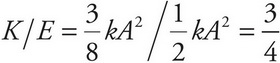# AP Physics 1 Question 247: Answer and Explanation

### Test Information

Question: 247

2. A block attached to an ideal spring undergoes simple harmonic motion about its equilibrium position (x = 0) with amplitude A. What fraction of the total energy is in the form of kinetic energy when the block is at position x =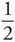A ?

• A.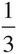• B.• C.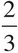• D.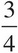Explanation:

D

By Conservation of Mechanical Energy, K + US is a constant for the motion of the block. At the endpoints of the oscillation region, the block's displacement, x, is equal to ±A. Since K = 0 here, all the energy is in the form of potential energy of the spring,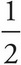kA2. BecausekA2 gives the total energy at these positions, it also gives the total energy at any other position.

Using the equation US(x) =kx2, find that, at x =A.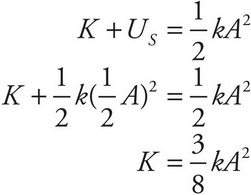Therefore,